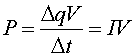Energy dissipation in resistors

As a charge q moves through a resistor, it loses a potential energy qV where V is the potential drop across the resistor. This energy goes into heat, much like the way a ball of putty that falls off a cliff converts its potential energy to heat when it hits the ground. We refer to this conversion of potential energy into heat as dissipation.

The power dissipated in a resistor is the energy dissipated per time. If an amount of charge Dq moves through the resistor in a time Dt, the power loss iswhere I is the current through the resistor and V is the voltage drop across it.

The formula P = I V also gives the power generated by a battery if I is the current coming from the battery and V is its voltage.